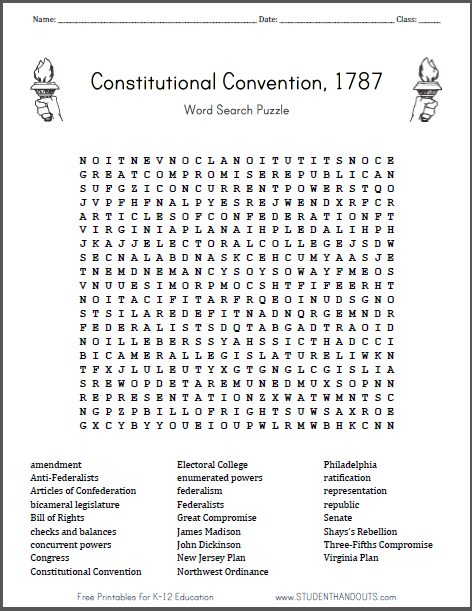Constitutional Convention Word Search Puzzle | Student Handouts
 Share the learning joy!

Constitutional Convention PuzzleClick here to print this word search puzzle worksheet on the Constitutional Convention (1787). The answer key is located below. For more of our free worksheets on this topic, click here.

Puzzle Terms: amendment, Anti-Federalists, Articles of Confederation, bicameral legislature, Bill of Rights, checks and balances, concurrent powers, Congress, Constitutional Convention, Electoral College, enumerated powers, federalism, Federalists, Great Compromise, James Madison, John Dickinson, New Jersey Plan, Northwest Ordinance, Philadelphia, ratification, representation, republic, Senate, Shays’s Rebellion, Three-Fifths Compromise, Virginia Plan
N O I T N E V N O C L A N O I T U T I T S N O C +
G R E A T C O M P R O M I S E R E P U B L I C + N
+ + + + + + C O N C U R R E N T P O W E R S + + O
+ + + + + + N A L P Y E S R E J W E N + + + + + R
A R T I C L E S O F C O N F E D E R A T I O N + T
V I R G I N I A P L A N A I H P L E D A L I H P H
+ + + + + E L E C T O R A L C O L L E G E J S + W
S E C N A L A B D N A S K C E H C + + + + A S J E
T N E M D N E M A + + + + + + + + + + + F M E O S
+ + + + E S I M O R P M O C S H T F I F E E R H T
N O I T A C I F I T A R + + + E + + + + D S G N O
S T S I L A R E D E F I T N A + N + + + E M N D R
F E D E R A L I S T S + + + + + + A + + R A O I D
N O I L L E B E R S S Y A H S + + + T + A D C C I
B I C A M E R A L L E G I S L A T U R E L I + K N
+ + + + + + + + + + + + + + + + + + + + I S + I A
S R E W O P D E T A R E M U N E + + + + S O + N N
R E P R E S E N T A T I O N + + + + + + M N + S C
+ + + + + B I L L O F R I G H T S + + + + + + O E
+ + + + + + + + + + + + + + + + + + + + + + + N +